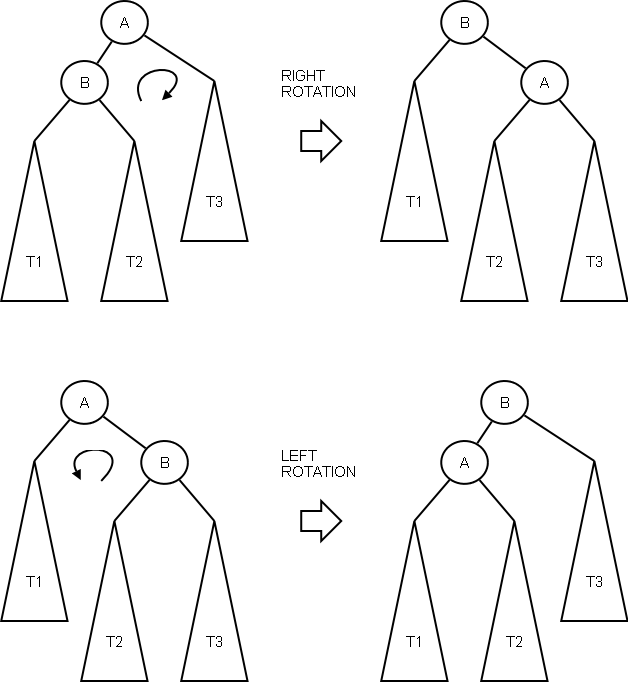# 算法基础笔记0x06 —— 二叉搜索

Dictionary also called dynamic set, which support the following operations.

• find(k) returns 1 if k is in D, 0 otherwise.
• insert(k) puts k in D.
• delete(k) removes k from D.
• pred(k) / succ(k) return the predecessor / successor to k in D, resp.
• min / max return the minimum / maximum value in D, resp.

Ways to implement a dictionary.

Linked list O(n) n: # of items, slow
Hash table O(1) expected for find, insert, delete. O(n) for pred / succ and min / max. Randomized algorithm. O(n) in worst case.
Balanced search tree O(logn) Many variant, AVL, red-black, B-tree
Skip lists, splay trees, etc. O(logn) Additional functionalities, like parallelizable, faster to access recent items, etc.

## Binary Search Tree• In a binary search tree, each node x has at most two children, the left and right child, denoted x.l and x.r.
• The node also stores a key, denoted x.key.
• BST Property For any node x, keys in left subtree of x are all less than x.key, and keys in right subtree are all bigger than x.key.

### Basic Operations

Find Operation

• To find whether k is in the tree, start at the root and move downwards.
• At node x, compare k’=x.key and k.
• If k=k’, return node x.
• If k<k’, then if k is in tree, it must be left of x. So go to x’s left child.
• Else k>k’, and go to x’s right child.
• Once reach a leaf with key k’, if k’ = k, then we found the key.
• If k≠k’, k is not in the tree, so output FALSE.

Insertion Operation

• To insert key k into tree, first do find(k) to get to a leaf.
• If find returns a node, then k already in D, so stop.
• Otherwise, if find returns node x.
• Let k’=x.key.
• If k<k’, make a left child for x, and set its key to k.
• Else make right child for x, and set its right key to k.

Find Successors

• The successor to a key k is the smallest key in D larger than k.
• Ex succ(4) = 6, succ(10) = 11.
• Assume k is in D. To find k’s successor, first find node x with key k.
• If x has no right child, then
• If x is a left child, x’s parent is its successor.
• Ex succ(3) = 4
• If x is a right child, keep following parent pointers until an ancestor has a bigger key.
• Ex succ(9) = 10
• Otherwise x has a right child.
• Go to the right child, then keep following left children till reach a leaf.
• Ex succ(10) = 11.

Deletion Operaiton

• First, do a find(k) to get to a node x.
• If find(k) failed, stop.
• Otherwise, consider whether x has 0, 1 or 2 children.
• 0 children Just remove x.
• 1 child Remove x, connect x’s child to x’s parent.
• 2 children First, find the successor k’ of k.
• Since x has a right child, we’re in case 3 of the successor operation.
• k’ has no left child.
• Consider whether k’ is right child of k.
• Case 1 If k’ is right child of k, then replace k by k’.
• Case 2 Otherwise, replace k by k’, and replace k’ by its right child.
• 若删除元素有两个元素，找到删除元素的下一个元素(successor)并用其将删除元素替换

### Balance and Complexity

• It’s too costly to make all nodes completely balanced.
• We settle for nearly balanced trees, where for all nodes x: |ht(x.r)-ht(x.l)| ≤ 1, where ht(z) is height of a node z.
• For each node x, let bal(x) = ht(x.r) – ht(x.l).

### Rotations – Fix Imbalance

If a node is imbalanced, then rotating at the node can improve its balance.
Two types, left and right rotation.## AVL Tree

• AVL tree is a BST where the balance at all nodes is -1, 0 or 1.
• Call this the AVL invariant.

### Operatioins

Insertion operation

• After insertion, if all nodes have balance 0, 1 or -1, done.
• Otherwise, find the lowest ±2 balance node x on the path from the inserted node to the root.
• Lemma After the rotation(s), all nodes outside of x’s subtree also have balance 1, -1 or 0.

Deletion operation

• After deletion, again find lowest ±2 balance node x, if any.
• Lemma After the rotation(s), ht(x) decreases by at most 1.

### Complexity

• Thm An AVL tree with n nodes has O(logn) height.
• Proof Let s(h) be the minimum number of nodes in an AVL tree with height h.
• s(1)=1, s(2)=2.
• For h≥3, the root of the AVL tree has one subtree with height h-1, and another subtree with height ≥ h-2, by the AVL invariant.
• Both subtrees are AVL trees, so s(h) ≥ 1 + s(h-1) + s(h-2).
• Also, s(h-1) ≥ s(h-2).
• So s(h) ≥ $2^{h/2}$.
• So if there are n nodes in the AVL tree, n ≥ $2^{h/2}$, and so h=O(logn)
• Finding, inserting and deleting keys from an AVL tree with n nodes take O(logn) time.

Other balanced trees

• RB trees also use rotations to maintain approximate balance, but have looser balance conditions.
• Advantage of RB trees is that they require only O(1) rotations for insertion and deletions.
• AVL trees require O(1) rotations for insertions, but O(logn) rotations for deletions.
• However, AVL trees have tighter bounds on height.
• AVL tree with n nodes has height ≤ $1.44log_2{n}$.
• RB tree with n nodes has height ≤ $2log_2{n}$.
• So AVL trees are faster for search intensive workloads, whereas RB trees are better for more dynamic BSTs.